Theory and Modern Applications

# Solving a system of fractional partial differential equations arising in the model of HIV infection of CD4+ cells and attractor one-dimensional Keller-Segel equations

## Abstract

In this paper, we make use of the relatively new analytical technique, the homotopy decomposition method (HDM), to solve a system of fractional nonlinear differential equations that arise in the model for HIV infection of CD4+ T cells and attractor one-dimensional Keller-Segel equations. The technique is described and illustrated with a numerical example. The reliability of HDM and the reduction in computations give HDM a wider applicability. In addition, the calculations involved in HDM are very simple and straightforward.

## 1 Introduction

Fractional calculus has been used to model physical and engineering processes, which are found to be best described by fractional differential equations . It is worth nothing that the standard mathematical models of integer-order derivatives, including nonlinear models, do not work adequately in many cases . In the recent years, fractional calculus has played a very important role in various fields such as mechanics, electricity, chemistry, biology, economics, notably control theory, and signal and image processing . Major topics include anomalous diffusion, vibration and control, continuous time random walk, Levy statistics, fractional Brownian motion, fractional neutron point kinetic model, power law, Riesz potential, fractional derivative and fractals, computational fractional derivative equations, nonlocal phenomena, history-dependent process, porous media, fractional filters, biomedical engineering, fractional phase-locked loops, fractional variational principles, fractional transforms, fractional wavelet, fractional predator-prey system, soft matter mechanics, fractional signal and image processing, singularities analysis and integral representations for fractional differential systems, special functions related to fractional calculus, non-Fourier heat conduction, acoustic dissipation, geophysics, relaxation, creep, viscoelasticity, rheology, fluid dynamics, and chaos. An excellent literature of this can be found in .

The purpose of this paper is to make use of the homotopy decomposition method (HDM) [11, 12], a relatively new analytical technique, to solve a system of fractional partial differential equations that arise in the model for HIV infection of CD4+ T cells and attractor one-dimensional Keller-Segel equations. In this study, we consider a fractional case of the HIV infection model of CD4+ T cells examined in . This model is given by the basic three components which are the concentration of susceptible CD4+ T cells, CD4+ T cells infected by the HIV viruses and free HIV virus particles in the blood. These CD4+ T cells with order cells in human immunity systems fight against diseases. HIV use cells in order to propagate. In a healthy person, the number of CD4+ T cells is 800/1200 mm3. CD4+ T cells are also called leukocytes or T helper cells on one hand. On the other hand, we consider the fractional parabolic systems to describe the aggregation process of cellular slime mold by the chemical attraction . Recently, the Keller-Segel (KS) equations attracted interest of many mathematicians. Local solutions were studied by the second author .

The paper is structured as follows. In Section 2, we give a brief history and properties of the fractional derivative order definition. In Section 3, we present the basic ideal of the homotopy decomposition method for solving fractional partial differential equations. We present the application of the HDM for a system of fractional nonlinear differential equations and numerical results in Section 4. The conclusions are then given in the final Section 5.

## 2 Fractional order derivative

### 2.1 Brief history

There exists a vast literature on different definitions of fractional derivatives. The most popular ones are the Riemann-Liouville and the Caputo derivatives. For Caputo, we have

${}_{0}{}^{C}D_{x}^{\alpha }\left(f\left(x\right)\right)=\frac{1}{\mathrm{\Gamma }\left(n-\alpha \right)}{\int }_{0}^{x}{\left(x-t\right)}^{n-\alpha -1}\frac{{d}^{n}f\left(t\right)}{d{t}^{n}}\phantom{\rule{0.2em}{0ex}}dt.$
(2.1)

For the case of Riemann-Liouville, we have the following definition:

${D}_{x}^{\alpha }\left(f\left(x\right)\right)=\frac{1}{\mathrm{\Gamma }\left(n-\alpha \right)}\frac{{d}^{n}}{d{x}^{n}}{\int }_{0}^{x}{\left(x-t\right)}^{n-\alpha -1}f\left(t\right)\phantom{\rule{0.2em}{0ex}}dt.$
(2.2)

Each fractional derivative presents some advantages and disadvantages [16, 17]. The Riemann-Liouville derivative of a constant is not zero, while the Caputo derivative of a constant is zero but demands higher conditions of regularity for differentiability: to compute the fractional derivative of a function in the Caputo sense, we must first calculate its derivative. Caputo derivatives are defined only for differentiable functions, while functions that have no first-order derivative might have fractional derivatives of all orders less than one in the Riemann-Liouville sense [18, 19]. Recently, Guy Jumarie (see [20, 21]) proposed a simple alternative definition to the Riemann-Liouville derivative

${D}_{x}^{\alpha }\left(f\left(x\right)\right)=\frac{1}{\mathrm{\Gamma }\left(n-\alpha \right)}\frac{{d}^{n}}{d{x}^{n}}{\int }_{0}^{x}{\left(x-t\right)}^{n-\alpha -1}\left\{f\left(t\right)-f\left(0\right)\right\}\phantom{\rule{0.2em}{0ex}}dt.$
(2.3)

His modified Riemann-Liouville derivative seems to have advantages over both the standard Riemann-Liouville and the Caputo fractional derivatives: it is defined for arbitrary continuous (non-differentiable) functions, and the fractional derivative of a constant is equal to zero.

We can point out that Caputo and Riemann-Liouville derivatives may have their disadvantages, but they are more useful when dealing with some real world problems . Every definition must be used accordingly.

### 2.2 Properties and definitions

Definition 1 A real function $f\left(x\right)$, $x>0$, is said to be in the space ${C}_{\mu }$, $\mu \in \mathbb{R}$ if there exists a real number $p>\mu$, such that $f\left(x\right)={x}^{p}h\left(x\right)$, where $h\left(x\right)\in C\left[0,\mathrm{\infty }\right)$, and it is said to be in the space ${C}_{\mu }^{m}$ if ${f}^{\left(m\right)}\in {C}_{\mu }$, $m\in \mathbb{N}$.

Definition 2 The Riemann-Liouville fractional integral operator of order $\alpha \ge 0$, of a function $f\in {C}_{\mu }$, $\mu \ge -1$, is defined as

$\begin{array}{r}{J}^{\alpha }f\left(x\right)=\frac{1}{\mathrm{\Gamma }\left(\alpha \right)}{\int }_{0}^{x}{\left(x-t\right)}^{\alpha -1}f\left(t\right)\phantom{\rule{0.2em}{0ex}}dt,\phantom{\rule{1em}{0ex}}\alpha >0,x>0,\\ {J}^{0}f\left(x\right)=f\left(x\right).\end{array}$
(2.4)

Properties of the operator can be found in [18, 19], we mention only the following.

For $f\in {C}_{\mu }$, $\mu \ge -1$, $\alpha ,\beta \ge 0$ and $\gamma >-1$:

$\begin{array}{r}{J}^{\alpha }{J}^{\beta }f\left(x\right)={J}^{\alpha +\beta }f\left(x\right),\\ {J}^{\alpha }{J}^{\beta }f\left(x\right)={J}^{\beta }{J}^{\alpha }f\left(x\right),\\ {J}^{\alpha }{x}^{\gamma }=\frac{\mathrm{\Gamma }\left(\gamma +1\right)}{\mathrm{\Gamma }\left(\alpha +\gamma +1\right)}{x}^{\alpha +\gamma }.\end{array}$
(2.5)

Lemma 1 If $m-1<\alpha \le m$, $m\in \mathbb{N}$ and $f\in {C}_{\mu }^{m}$, $\mu \ge -1$, then

${D}^{\alpha }{J}^{\alpha }f\left(x\right)=f\left(x\right)\phantom{\rule{1em}{0ex}}\mathit{\text{and}}\phantom{\rule{1em}{0ex}}{J}^{\alpha }{D}_{0}^{\alpha }f\left(x\right)=f\left(x\right)-\sum _{k=0}^{m-1}{f}^{\left(k\right)}\left({0}^{+}\right)\frac{{x}^{k}}{k!},\phantom{\rule{1em}{0ex}}x>0.$
(2.6)

Definition 3 (Partial derivatives of fractional order)

Assume now that $f\left(x\right)$ is a function of n variables ${x}_{i}$, $i=1,\dots ,n$, which is also of class C on $D\in {\mathbb{R}}_{n}$. We define a partial derivative of order α for f with respect to ${x}_{i}$

$a{\partial }_{\underline{x}}^{\alpha }f=\frac{1}{\mathrm{\Gamma }\left(m-\alpha \right)}{{\int }_{a}^{{x}_{i}}{\left({x}_{i}-t\right)}^{m-\alpha -1}{\partial }_{xi}^{m}f\left({x}_{j}\right)|}_{xj=t}\phantom{\rule{0.2em}{0ex}}dt.$
(2.7)

If it exists, where ${\partial }_{xi}^{m}$ is the usual partial derivative of integer order m.

## 3 Basic idea of the HDM [11, 12]

To illustrate the basic idea of this method, we consider a general nonlinear non-homogeneous fractional partial differential equation with initial conditions of the following form:

$\frac{{\partial }^{\alpha }U\left(x,t\right)}{\partial {t}^{\alpha }}=L\left(U\left(x,t\right)\right)+N\left(U\left(x,t\right)\right)+f\left(x,t\right),\phantom{\rule{1em}{0ex}}\alpha >0,$
(3.1)

subject to the initial conditions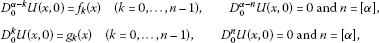where $\frac{{\partial }^{\alpha }}{\partial {t}^{\alpha }}$ denotes the Caputo or Riemann-Liouville fraction derivative operator, f is a known function, N is the general nonlinear fractional differential operator and L represents a linear fractional differential operator. The first step of the method here is to transform the fractional partial differential equation to the fractional partial integral equation by applying the inverse operator $\frac{{\partial }^{\alpha }}{\partial {t}^{\alpha }}$ on both sides of equation (3.1) to obtain: in the case of the Riemann-Liouville fractional derivative,

$\begin{array}{rcl}U\left(x,t\right)& =& \sum _{j=1}^{n-1}\frac{{f}_{j}\left(x\right)}{\mathrm{\Gamma }\left(\alpha -j+1\right)}{t}^{\alpha -j}\\ +\frac{1}{\mathrm{\Gamma }\left(\alpha \right)}{\int }_{0}^{t}{\left(t-\tau \right)}^{\alpha -1}\left[L\left(U\left(x,\tau \right)\right)+N\left(U\left(x,\tau \right)\right)+f\left(x,\tau \right)\right]\phantom{\rule{0.2em}{0ex}}d\tau ;\end{array}$
(3.2)

in the case of the Caputo fractional derivative,

$U\left(x,t\right)=\sum _{j=1}^{n-1}\frac{{g}_{j}\left(x\right)}{\mathrm{\Gamma }\left(\alpha -j+1\right)}{t}^{\alpha -j}+\frac{1}{\mathrm{\Gamma }\left(\alpha \right)}{\int }_{0}^{t}{\left(t-\tau \right)}^{\alpha -1}\left[L\left(U\left(x,\tau \right)\right)+N\left(U\left(x,\tau \right)\right)+f\left(x,\tau \right)\right]\phantom{\rule{0.2em}{0ex}}d\tau .$

Or, in general, by putting

$\sum _{j=1}^{n-1}\frac{{f}_{j}\left(x\right)}{\mathrm{\Gamma }\left(\alpha -j+1\right)}{t}^{\alpha -j}=f\left(x,t\right)\phantom{\rule{1em}{0ex}}\text{or}\phantom{\rule{1em}{0ex}}f\left(x,t\right)=\sum _{j=1}^{n-1}\frac{{g}_{j}\left(x\right)}{\mathrm{\Gamma }\left(\alpha -j+1\right)}{t}^{j},$

we obtain

$U\left(x,t\right)=T\left(x,t\right)+\frac{1}{\mathrm{\Gamma }\left(\alpha \right)}{\int }_{0}^{t}{\left(t-\tau \right)}^{\alpha -1}\left[L\left(U\left(x,\tau \right)\right)+N\left(U\left(x,\tau \right)\right)+f\left(x,\tau \right)\right]\phantom{\rule{0.2em}{0ex}}d\tau .$
(3.3)

In the homotopy decomposition method, the basic assumption is that the solutions can be written as a power series in p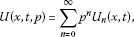(3.4a)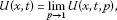(3.4b)

and the nonlinear term can be decomposed as

$NU\left(x,t\right)=\sum _{n=0}^{\mathrm{\infty }}{p}^{n}{\mathcal{H}}_{n}\left(U\right),$
(3.5)

where $p\in \left(0,1\right]$ is an embedding parameter. ${\mathcal{H}}_{n}\left(U\right)$ is He’s polynomials that can be generated by

${\mathcal{H}}_{n}\left({U}_{0},\dots ,{U}_{n}\right)=\frac{1}{n!}\frac{{\partial }^{n}}{\partial {p}^{n}}\left[N\left(\sum _{j=0}^{\mathrm{\infty }}{p}^{j}{U}_{j}\left(x,t\right)\right)\right],\phantom{\rule{1em}{0ex}}n=0,1,2,\dots .$
(3.6)

The homotopy decomposition method is obtained by the graceful coupling of homotopy technique with the Abel integral and is given by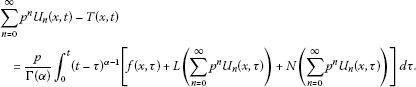(3.7)

Comparing the terms of the same powers of p gives solutions of various orders with the first term

${U}_{0}\left(x,t\right)=T\left(x,t\right).$
(3.8)

Theorem ()

Suppose that X and Y are the Banach spaces and $V:X\to Y$ is a contraction nonlinear mapping. If the sequence generated by the homotopy decomposition method is regarded as

${U}_{n}\left(x,t\right)=V\left({U}_{n-1}\left(x,t\right)\right)=\sum _{i=0}^{n-1}{U}_{i}\left(x,t\right),\phantom{\rule{1em}{0ex}}n=1,2,3\dots ,$

then the following statements hold:

1. (1)

$\parallel {U}_{n}\left(x,t\right)-U\left(x,t\right)\parallel \le {\phi }^{n}\parallel T\left(x,t\right)-U\left(x,t\right)\parallel$;

2. (2)

${U}_{n}\left(x,t\right)$ is always in the neighbourhood of $U\left(x,t\right)$ meaning ${U}_{n}\left(x,t\right)\in B\left(U\left(x,t\right),r\right)=\left\{{U}^{\ast }\left(x,t\right)/\parallel {U}^{\ast }\left(x,t\right)-U\left(x,t\right)\parallel \right\}$;

3. (3)

${lim}_{n\to \mathrm{\infty }}{U}_{n}\left(x,t\right)=U\left(x,t\right)$.

Proof (1) We prove the statement (1) by induction on n, $\parallel {U}_{1}-U\parallel =\parallel G\left({U}_{0}\right)-U\parallel$, and according to the Banach fixed point theorem, V has a fixed point U meaning $V\left(U\right)=U$; therefore,

$\parallel {U}_{1}-U\parallel =\parallel G\left({U}_{0}\right)-U\parallel =\parallel G\left({U}_{0}\right)-G\left(U\right)\parallel \le \phi \parallel {U}_{0}-U\parallel =\phi \parallel T\left(x,t\right)-U\parallel$

since V is a contraction mapping.

Assume that $\parallel {U}_{n-1}-U\parallel \le {\phi }^{n-1}\parallel T\left(x,0\right)-U\left(x,t\right)\parallel$ is an induction hypothesis, then

$\parallel {U}_{n}-U\parallel =\parallel G\left({U}_{n-1}\right)-G\left(U\right)\parallel \le \phi \parallel {U}_{n-1}-U\parallel \le \phi {\phi }^{n-1}\parallel T\left(x,t\right)-U\parallel .$
1. (2)

The first concern here is to prove that $T\left(x,t\right)\in B\left(U\left(x,t\right),r\right)$, and this is achieved by induction on m. So, for $m=1$, $\parallel T\left(x,t\right)-U\left(x,t\right)\parallel =\parallel U\left(x,0\right)-U\left(x,t\right)\parallel \le r$ with $U\left(x,0\right)$ the initial condition.

Assume that $\parallel T\left(x,t\right)-U\left(x,t\right)\parallel \le r$ for $m-2$ is an induction hypothesis, then

$\begin{array}{rcl}\parallel T\left(x,t\right)-U\left(x,t\right)\parallel & =& \parallel {T}_{m-2}\left(x,t\right)-\frac{{f}_{m}\left(x\right)}{\mathrm{\Gamma }\left(\alpha -m+1\right)}{t}^{\alpha -m}\parallel \\ \le & \parallel {T}_{m-1}\left(x,t\right)-U\left(x,t\right)\parallel +\parallel \frac{{f}_{m}\left(x\right)}{\mathrm{\Gamma }\left(\alpha -m+1\right)}{t}^{\alpha -m}\parallel \\ =& r.\end{array}$

Now, for all $n\ge 1$, using (1) we have

$\parallel {U}_{n}-U\parallel \le {\phi }^{n}\parallel T\left(x,t\right)-U\parallel \le {\phi }^{n}r\le r.$
1. (3)

Using (2) and the fact that ${lim}_{n\to \mathrm{\infty }}{\phi }^{n}=0$ yields that ${lim}_{n\to \mathrm{\infty }}\parallel {U}_{n}-U\parallel =0$; therefore,

$\underset{n\to \mathrm{\infty }}{lim}{U}_{n}=U.$

□

## 4 Application

‘In learning science examples are more useful than rules’ (Isaac Newton). In this section we apply this method to solving a system of fractional differential equations.

### 4.1 Fractional model for HIV infection of CD4+ T

This model is characterized by the system of nonlinear differential equations

$\left\{\begin{array}{c}\frac{{d}^{\mu }T}{d{t}^{\mu }}=p-\alpha T+rT\left(1-\frac{T+I}{{T}_{\mathrm{max}}}\right)-kVT,\hfill \\ \frac{{d}^{\eta }I}{d{t}^{\eta }}=kVT-\beta I,\phantom{\rule{1em}{0ex}}0<\mu ,\eta ,\upsilon \le 1,\hfill \\ \frac{{d}^{\upsilon }V}{d{t}^{\upsilon }}=N\beta I-\gamma V\hfill \end{array}$
(4.1)

subject to the initial conditions

$T\left(0\right)={T}_{0},\phantom{\rule{2em}{0ex}}I\left(0\right)={I}_{0},\phantom{\rule{2em}{0ex}}V\left(0\right)={V}_{0}.$
(4.2)

Here, r is any positive constant; $T\left(t\right)$, $I\left(t\right)$ and $V\left(t\right)$ show the concentration of susceptible CD4+ T cells, CD4+ T cells infected by the HIV viruses and free HIV virus particles in the blood, respectively; α, β and γ stand for natural turnover rates of uninfected T cells, infected T cells and virus particles, respectively; $\left(1-\frac{T+I}{{T}_{\mathrm{max}}}\right)$ describes the logistic growth of healthy CD4+ T cells, and proliferation of infected CD4+ T cells is neglected. For $k>0$ is the infection rate, the term KVT describes the incidence of HIV infection of healthy CD4+ T cells. Each infected CD4+ T cell is assumed to produce one virus particle during its lifetime, including any of its daughter cells. The body is believed to produce CD4+ T cells from precursors in the bone marrow and thymus at a constant rate p. T cells multiply through mitosis with a rate r when T cells are stimulated by antigen or mitogen. ${T}_{\mathrm{max}}$ denotes the maximum CD4+ T cell concentration in the body . We chose the following initial condition and parameters as in :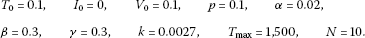Following the HDM steps, we arrive at the following integral equations: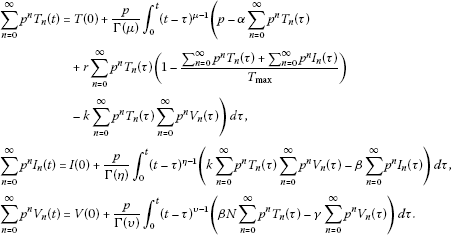(4.3)

Comparing the terms of the same power of p, we obtain the following integral equations that are very easy to solve. Note that, with the homotopy perturbation method (HPM), one will obtain a set of ordinary differential equations after comparing the terms of the same power of p, which is very hard to compute in the case of high order ODE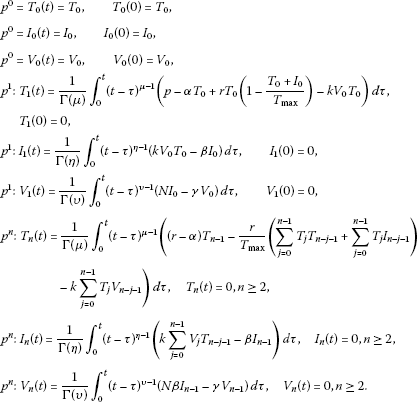(4.4a)

The components of the series solution are obtained directly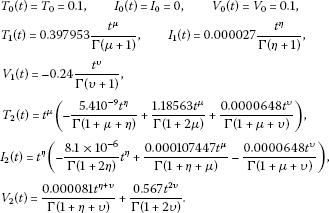(4.4b)

Using the package Mathematica, in the same manner one can obtain the rest of the components. But here, a few terms were computed and the asymptotic solution is given by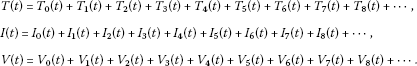(4.5)

Figures 1-4 show the graphical representation of the approximated solutions obtained via HDM for $\mu =0.9$, $\eta =0.99$ and $\upsilon =1$. It is worth nothing that the solution of the fractional system is not only a function of time, but also a continuous function of fractional order derivatives υ and η. The new parameters (μ, υ and η) introduced in the model can be viewed as new physical parameters that characterise the interaction between the concentration of susceptible CD4+ T cells, CD4+ T cells infected by the HIV viruses and free HIV virus particles in the blood.

### 4.2 Fractional attractor one-dimensional Keller-Segel equations

In 1970 Keller and Segel  presented parabolic systems to describe the aggregation process of cellular slime mold by chemical attraction. The system of a simplified form in the one-dimensional case is written as follows:

$\begin{array}{r}\frac{\partial u\left(x,t\right)}{\partial t}=a\frac{{\partial }^{2}u\left(x,t\right)}{\partial {x}^{2}}-\frac{\partial }{\partial x}\left(u\left(x,t\right)\frac{\partial \chi \left(\rho \right)}{\partial x}\right),\\ \frac{\partial \rho \left(x,t\right)}{\partial t}=b\frac{{\partial }^{2}\rho \left(x,t\right)}{\partial {x}^{2}}+cu\left(x,t\right)-d\rho \left(x,t\right)\end{array}$
(4.6)

subject to the boundary condition

$\frac{\partial u\left(\alpha ,t\right)}{\partial x}=\frac{\partial u\left(\beta ,t\right)}{\partial x}=\frac{\partial \rho \left(\alpha ,t\right)}{\partial x}=\frac{\partial \rho \left(\beta ,t\right)}{\partial x}=0,$
(4.7)

and the initial conditions

$u\left(x,0\right)={u}_{0}\left(x\right),\phantom{\rule{2em}{0ex}}\rho \left(x,0\right)={\rho }_{0}\left(x\right),\phantom{\rule{1em}{0ex}}x\in I.$
(4.8)

Here, $I=\left(\alpha ,\beta \right)$ is a bounded open interval, a, b, c and d are positive constants. The unknown functions $u\left(x,t\right)$ and $\rho \left(x,t\right)$ denote the concentration of amoebae and the concentration of a chemical substance, respectively, in $I×\left(0,\mathrm{\infty }\right)$. The chemotactic term $\frac{\partial }{\partial x}\left(u\left(x,t\right)\frac{\partial \chi \left(\rho \right)}{\partial x}\right)$ indicates that the cells are sensitive to the chemicals and are attracted by them. $\chi \left(\rho \right)$, called the sensitivity function, is a smooth function of $\rho \in \left(0,\mathrm{\infty }\right)$ which describes a cell’s perception and response to the chemical stimulus ρ.

Example 1 Consider the following Keller-Segel equation with the sensitivity function $\chi \left(\rho \right)=1$.

Then the chemotactic term $\frac{\partial }{\partial x}\left(u\left(x,t\right)\frac{\partial \chi \left(\rho \right)}{\partial x}\right)=0$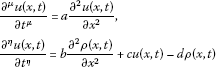subject to the initial conditions

$u\left(x,0\right)=m{e}^{-x2},\phantom{\rule{2em}{0ex}}\rho \left(x,0\right)=n{e}^{-x2},\phantom{\rule{1em}{0ex}}x>0.$
(4.9)

In view of the homotopy decomposition method, we obtain the following equations:

$\begin{array}{r}\sum _{n=0}^{\mathrm{\infty }}{p}^{n}{u}_{n}\left(x,t\right)-u\left(x,0\right)=\frac{p}{\mathrm{\Gamma }\left(\mu \right)}{\int }_{0}^{t}{\left(t-\tau \right)}^{\mu -1}a\frac{{\partial }^{2}}{\partial {x}^{2}}\left(\sum _{n=0}^{\mathrm{\infty }}{p}^{n}{u}_{n}\left(x,\tau \right)\right)\phantom{\rule{0.2em}{0ex}}d\tau ,\\ \sum _{n=0}^{\mathrm{\infty }}{p}^{n}{\rho }_{n}\left(x,t\right)+\rho \left(x,0\right)\\ \phantom{\rule{1em}{0ex}}=\frac{p}{\mathrm{\Gamma }\left(\eta \right)}{\int }_{0}^{t}{\left(t-\tau \right)}^{\eta -1}\left(b\frac{{\partial }^{2}}{\partial {x}^{2}}\left(\sum _{n=0}^{\mathrm{\infty }}{p}^{n}{\rho }_{n}\left(x,\tau \right)\right)\\ \phantom{\rule{1em}{0ex}}\phantom{\rule{1em}{0ex}}+c\sum _{n=0}^{\mathrm{\infty }}{p}^{n}{u}_{n}\left(x,\tau \right)-d\sum _{n=0}^{\mathrm{\infty }}{p}^{n}{\rho }_{n}\left(x,\tau \right)\right)\phantom{\rule{0.2em}{0ex}}d\tau .\end{array}$
(4.10)

Now, comparing the terms of the same power of p, we obtain the following integral equations: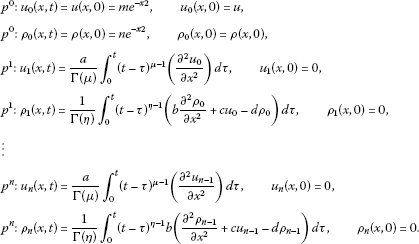(4.11a)

The following solutions are obtained: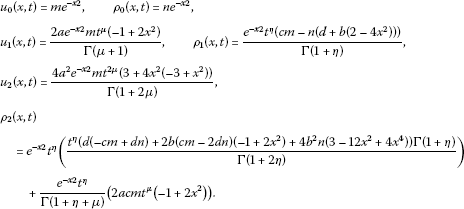(4.11b)

Using the software Mathematica, we can obtain the remaining terms. But here only a few terms of the series solutions are considered and the asymptotic solution is given as

$\begin{array}{r}u\left(x,t\right)={u}_{0}\left(x,t\right)+{u}_{1}\left(x,t\right)+{u}_{2}\left(x,t\right)+{u}_{3}\left(x,t\right)+\cdots ,\\ \rho \left(x,t\right)={\rho }_{0}\left(x,t\right)+{\rho }_{1}\left(x,t\right)+{\rho }_{2}\left(x,t\right)+{\rho }_{3}\left(x,t\right)+\cdots .\end{array}$
(4.11c)

Figures 5 and 6 show the biological behaviour of the coupled solution for the following set of theoretical parameters: $m=120$, $n=160$, $a=0.5$, $b=3$, $c=1$, and $d=2$, for a fixed distance $x=1$, $\mu =0.9$, $\eta =0.99$. It is worth noting that the solution of the fractional system is not only a function of time, but also a continuous function of fractional order derivatives. The new parameters (μ and η) introduced in the model can be viewed as new physical parameters that characterise the interaction between the concentration of amoebae and the concentration of a chemical substance.

Example 2 Consider the following Keller-Segel equation with the sensitivity function $\chi \left(\rho \right)=\rho$.

Then the chemotactic term $\frac{\partial }{\partial x}\left(u\left(x,t\right)\frac{\partial \chi \left(\rho \right)}{\partial x}\right)=\frac{\partial u\left(x,t\right)}{\partial x}\frac{\partial \rho \left(x,t\right)}{\partial x}+u\left(x,t\right)\frac{{\partial }^{2}\rho \left(x\right)}{\partial {x}^{2}}$

$\begin{array}{r}\frac{{\partial }^{\mu }u\left(x,t\right)}{\partial {t}^{\mu }}=a\frac{{\partial }^{2}u\left(x,t\right)}{\partial {x}^{2}}-\frac{\partial u\left(x,t\right)}{\partial x}\frac{\partial \rho \left(x,t\right)}{\partial x}+u\left(x,t\right)\frac{{\partial }^{2}\rho \left(x\right)}{\partial {x}^{2}},\\ \frac{{\partial }^{\eta }u\left(x,t\right)}{\partial {t}^{\eta }}=b\frac{{\partial }^{2}\rho \left(x,t\right)}{\partial {x}^{2}}+cu\left(x,t\right)+cu\left(x,t\right)-d\rho \left(x,t\right)\end{array}$
(4.12)

subject to the initial conditions

$u\left(x,0\right)={u}_{0}\left(x\right),\phantom{\rule{2em}{0ex}}\rho \left(x,0\right)={\rho }_{0}\left(x\right),\phantom{\rule{1em}{0ex}}x\in I.$
(4.13)

In view of the homotopy decomposition method, we arrive at the following set of integral equations that are very easy to handle: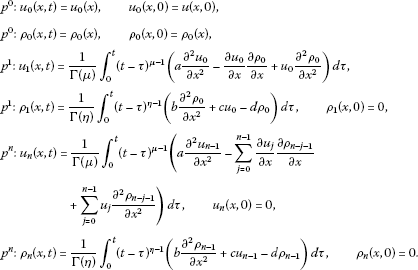(4.14)

The following solutions are read: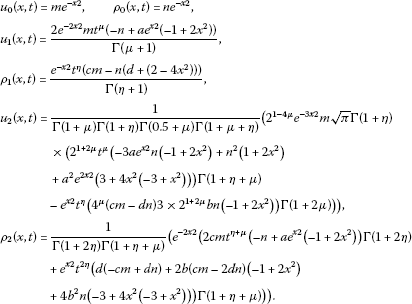(4.15)

Using the software Mathematica, the remaining terms can be obtained. But here only a few terms of the series solutions are considered and the asymptotic solution is given as

$\begin{array}{r}u\left(x,t\right)={u}_{0}\left(x,t\right)+{u}_{1}\left(x,t\right)+{u}_{2}\left(x,t\right)+{u}_{3}\left(x,t\right)+\cdots ,\\ \rho \left(x,t\right)={\rho }_{0}\left(x,t\right)+{\rho }_{1}\left(x,t\right)+{\rho }_{2}\left(x,t\right)+{\rho }_{3}\left(x,t\right)+\cdots .\end{array}$
(4.16)

## 5 Conclusions

Our concern was to provide asymptotic solutions to the system of fractional nonlinear differential equations that arise in the model for HIV infection of CD4+ T cells and attractor one-dimensional Keller-Segel equations, using a relatively new analytical technique, the homotopy decomposition method. We presented the brief history and some properties of the fractional derivative concept. It is demonstrated that HDM is a powerful and efficient tool for a system of FPDEs. In addition, the calculations involved in HDM are very simple and straightforward.

## References

1. Atangana A: Numerical solution of space-time fractional order derivative of groundwater flow equation. 2. International Conference of Algebra and Applied Analysis 2012, 20., Istanbul, June 20-24

2. Caputo M: Linear models of dissipation whose Q is almost frequency independent, part II. Geophys. J. Int. 1967, 13(5):529–539. 10.1111/j.1365-246X.1967.tb02303.x

3. Oldham KB, Spanier J: The Fractional Calculus. Academic Press, New York; 1974.

4. Podlubny I: Fractional Differential Equations. Academic Press, New York; 1999.

5. Baleanu D, Diethelm K, Scalas E, Trujillo JJ Series on Complexity, Nonlinearity and Chaos. In Fractional Calculus: Models and Numerical Methods. World Scientific, Singapore; 2012.

6. Kilbas AA, Srivastava HH, Trujillo JJ: Theory and Applications of Fractional Differential Equations. Elsevier, Amsterdam; 2006.

7. Miller KS, Ross B: An Introduction to the Fractional Calculus and Fractional Differential Equations. Wiley, New York; 1993.

8. Samko SG, Kilbas AA, Marichev OI: Fractional Integrals and Derivatives: Theory and Applications. Gordon & Breach, Yverdon; 1993.

9. Zaslavsky GM: Hamiltonian Chaos and Fractional Dynamics. Oxford University Press, London; 2005.

10. Perelson AS, Kirschner DE, Boer RD: Dynamics of HIV infection CD4+ T cells. Math. Biosci. 1993, 114: 81–125. 10.1016/0025-5564(93)90043-A

11. Atangana A, Secer A: The time-fractional coupled-Korteweg-de-Vries equations. Abstr. Appl. Anal. 2013., 2013: Article ID 947986. doi:10.1155/2013/947986

12. Atangana A, Botha JF: Analytical solution of groundwater flow equation via homotopy decomposition method. J. Earth Sci. Clim. Change 2012., 3: Article ID 1000115. doi:10.4172/2157–7617.1000115

13. Keller EF, Segel LA: Initiation of slime mold aggregation viewed as instability. J. Theor. Biol. 1970, 26: 399–415. 10.1016/0022-5193(70)90092-5

14. Yagi A: Norm homotopy of solutions to the parabolic system of chemotaxis. Math. Jpn. 1997, 45: 241–265.

15. Atangana A: New class of boundary value problems. Inf. Sci. Lett. 2012, 1(2):67–76.

16. Atangana A, Secer A: A note on fractional order derivatives and table of fractional derivative of some specials functions. Abstr. Appl. Anal. 2013., 2013: Article ID 279681. doi:10.1155/2013/279681

17. Podlubny I: Fractional Differential Equations. Academic Press, San Diego; 1999.

18. Samko SG, Kilbas AA, Marichev OI: Fractional Integrals and Derivatives. Gordon & Breach, Yverdon; 1993. Translated from the 1987 Russian original

19. Jumarie G:On the representation of fractional Brownian motion as an integral with respect to ${\left(\mathrm{d}t\right)}^{a}$. Appl. Math. Lett. 2005, 18(7):739–748. 10.1016/j.aml.2004.05.014

20. Atangana A, Ahmed A, Bıldık N: A generalized version of a low velocity impact between a rigid sphere and a transversely isotropic strain-hardening plate supported by a rigid substrate using the concept of noninteger derivatives. Abstr. Appl. Anal. 2013., 2013: Article ID 671321

21. Perelson AS, Nelson PW: Mathematical analysis of HIV-I dynamics in vivo. SIAM Rev. 1999, 41(1):3–44. 10.1137/S0036144598335107

22. Wang L, Li MY: Mathematical analysis of the global dynamics of a model for HIV infection of CD4+ T cells. Math. Biosci. 2006, 200: 44–57. 10.1016/j.mbs.2005.12.026

23. Asquith B, Bangham CRM: The dynamics of T-cell fratricide: application of a robust approach to mathematical modelling in immunology. J. Theor. Biol. 2003, 222: 53–69. 10.1016/S0022-5193(03)00013-4

24. Nowak M, May R: Mathematical biology of HIV infections: antigenic variation and diversity threshold. Math. Biosci. 1991, 106: 1–21. 10.1016/0025-5564(91)90037-J

25. Ongun MY: The Laplace Adomian decomposition method for solving a model for HIV infection of CD4+ T cells. Math. Comput. Model. 2011, 53: 597–603. 10.1016/j.mcm.2010.09.009

## Author information

Authors

### Corresponding author

Correspondence to Abdon Atangana.

### Competing interests

The authors declare that they have no competing interests.

### Authors’ contributions

AA wrote the first draft and EA corrected and improved the final version. Both authors read and approved the final draft.

## Authors’ original submitted files for images

Below are the links to the authors’ original submitted files for images.

## Rights and permissions

Open Access This article is distributed under the terms of the Creative Commons Attribution 2.0 International License (https://creativecommons.org/licenses/by/2.0), which permits unrestricted use, distribution, and reproduction in any medium, provided the original work is properly cited.

Reprints and Permissions

Atangana, A., Alabaraoye, E. Solving a system of fractional partial differential equations arising in the model of HIV infection of CD4+ cells and attractor one-dimensional Keller-Segel equations. Adv Differ Equ 2013, 94 (2013). https://doi.org/10.1186/1687-1847-2013-94

• Accepted:

• Published:

• DOI: https://doi.org/10.1186/1687-1847-2013-94

### Keywords

• model for HIV infection of CD4+ T cells
• attractor one-dimensional Keller-Segel equations
• fractional order derivative
• homotopy decomposition method